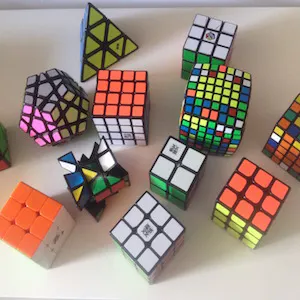# Electronic Safe with Arduino

Arduino Safe - 6 digit Passcode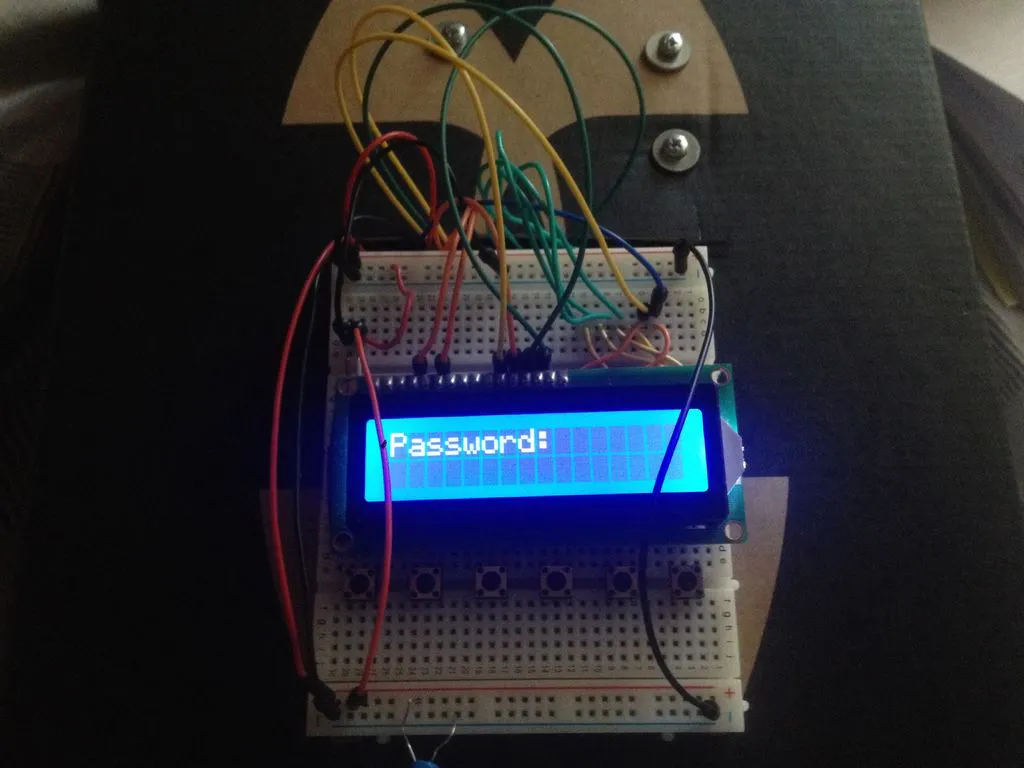## Things used in this project

### Hardware componentsJumper wires (generic)
×30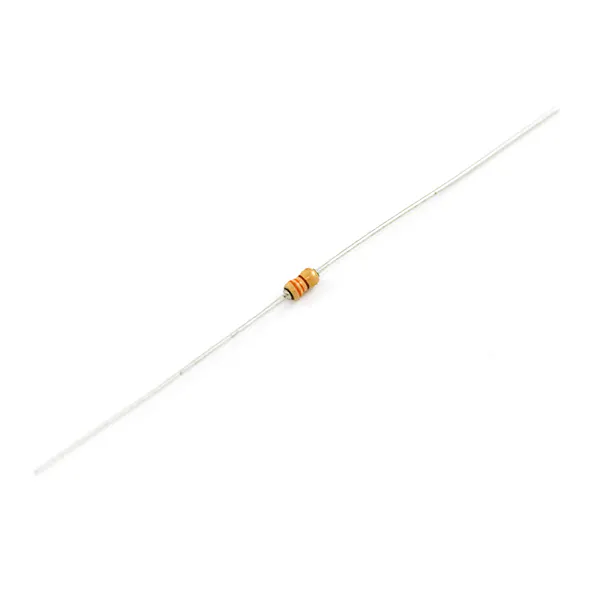Resistor 330 ohm
×1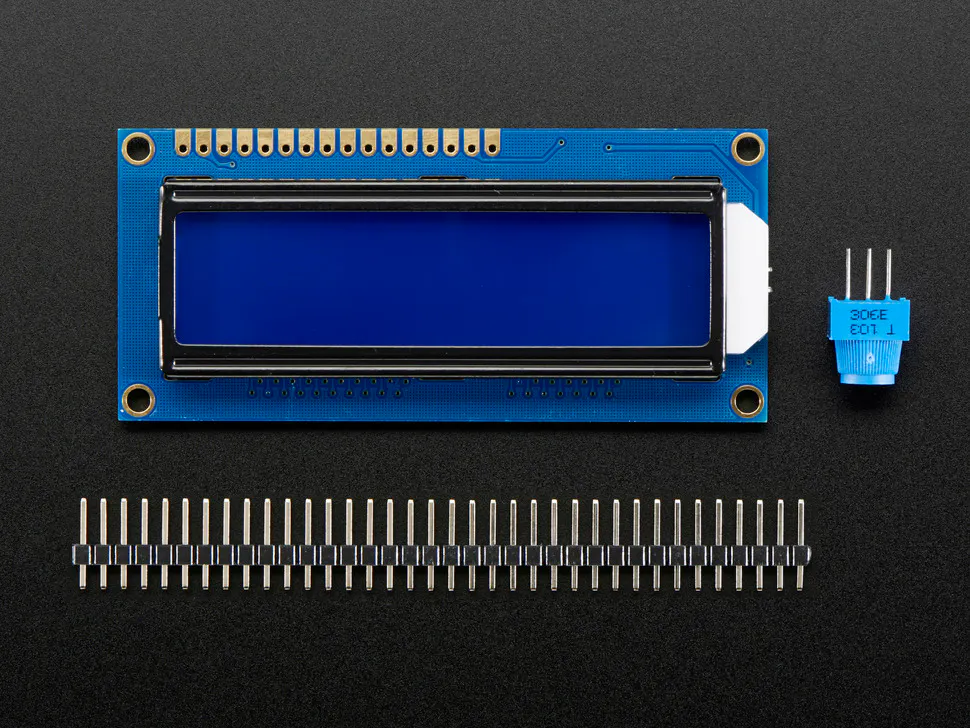Adafruit Standard LCD - 16x2 White on Blue
×1SparkFun Pushbutton switch 12mm
×6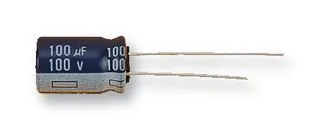Capacitor 100 µF
×1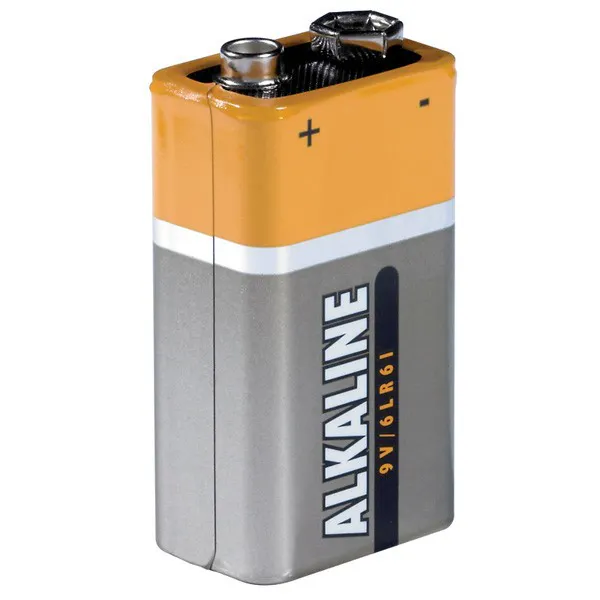9V battery (generic)
×1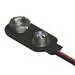9V Battery Clip
×1Arduino UNO & Genuino UNO
×1×1
 Servos (Tower Pro MG996R)
×1

### Software apps and online servicesArduino IDE

## Schematics

### Diagram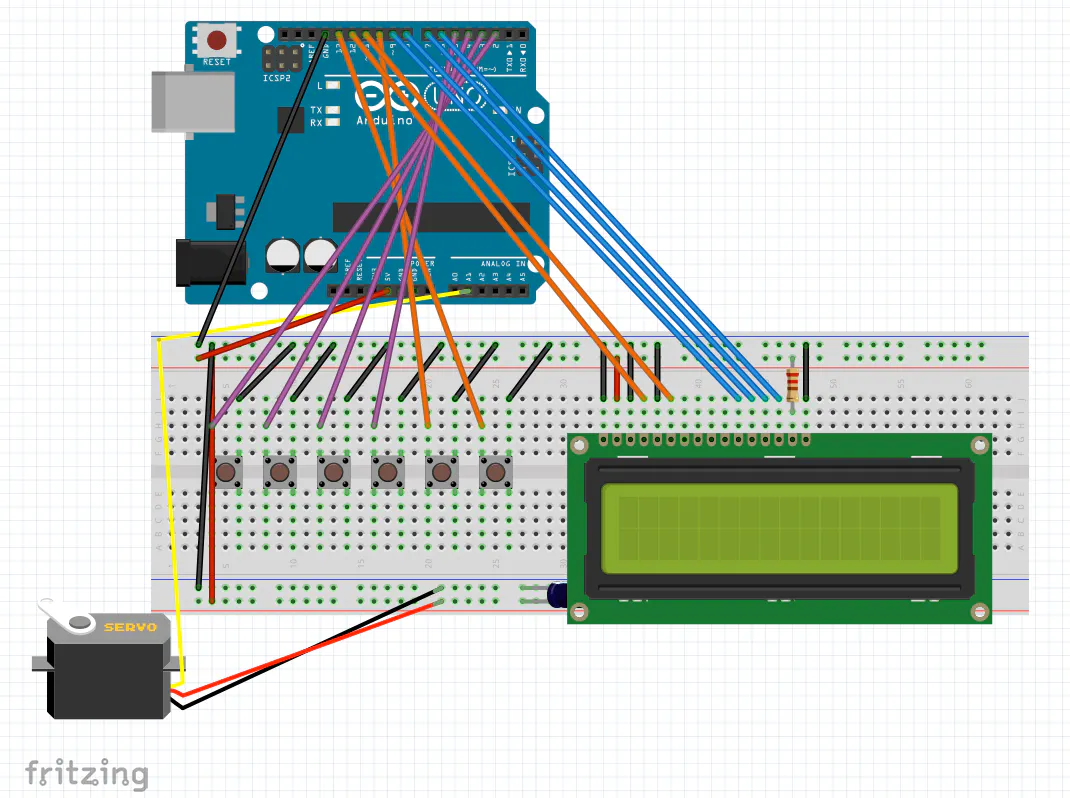## Code

### Code

Arduino
```/*
May 20, 2016
Contact me at: marcofusco111@gmail.com
*/

#include <LiquidCrystal.h>
#include <EEPROM.h>
#include <Servo.h>

static unsigned long SaveTimer;
static unsigned long SaveDelay = (30 * 1000);

char CODE = "1234E";
char Str;
char CodeLength = 4;
int Pos = 0;
bool Unlocked;
static unsigned long DisplayTimer;
static unsigned long DisplayDelay = 200;

LiquidCrystal lcd(12, 11, 9, 8, 7, 6);

int buttonPin1 = 2;
int buttonPin2 = 3;
int buttonPin3 = 4;
int buttonPin4 = 5;

int enterbutton = 10;
int clearlockbutton = 13;

Servo myServo;

void setup() {

myServo.attach(A1);

int EEPROMCodeOK = true;
for (Pos = 0; Pos <= (CodeLength); Pos++) {
if (!(strrchr("1123456789", Str[Pos]))) {
// not a valid code
EEPROMCodeOK = false;
}
}
Pos++;
if (Str[CodeLength + 1] != 'E') EEPROMCodeOK = false;
if (EEPROMCodeOK) {
Str[CodeLength + 2] = '\0';
strncpy(CODE, Str, CodeLength + 1);
}
ClearCode();

pinMode(buttonPin1, INPUT_PULLUP);
pinMode(buttonPin2, INPUT_PULLUP);
pinMode(buttonPin3, INPUT_PULLUP);
pinMode(buttonPin4, INPUT_PULLUP);

pinMode(enterbutton, INPUT_PULLUP);
pinMode(clearlockbutton, INPUT_PULLUP);

lcd.begin(16, 2);
lcd.setCursor(0, 0);
lcd.print("Hello.");
delay(2000);
lcd.clear();
lcd.setCursor(0, 0);

DisplayTimer = millis() + 200;
}

void loop() {

Lock();

Pos = constrain(Pos, 0, CodeLength);

lcd.setCursor(9, 0);

if (buttonState1 == LOW) {
Str[Pos] = '1';
Pos++;
Str[Pos] = '\0';
delay(250);

}

else if (buttonState2 == LOW) {
Str[Pos] = '2';
Pos++;
Str[Pos] = '\0';
delay(250);

}

else if (buttonState3 == LOW) {
Str[Pos] = '3';
Pos++;
Str[Pos] = '\0';
delay(250);
}

else if (buttonState4 == LOW) {
Str[Pos] = '4';
Pos++;
Str[Pos] = '\0';
delay(250);

}
else if (enterButtonState == LOW) {
Str[Pos] = 'E';
Pos++;
Str[Pos] = '\0';
delay(250);
if (strcmp (Str,CODE) == 0) {
Unlocked = true;
lcd.setCursor(0, 0);
lcd.print(" Access Granted");
delay(2000);
lcd.clear();
lcd.print("    Unlocked");
}
else if (SaveTimer > millis() && (Pos + 1) == CodeLength) {

strcpy(CODE, Str);
for (Pos = 0; Pos <= (CodeLength + 1); Pos++) {
EEPROM.write(Pos, Str[Pos]);
}
lcd.setCursor(0, 0);
lcd.print("Saving Code:");
lcd.setCursor(0, 1);
lcd.print(Str);

Unlocked = true;
}

else {

lcd.clear();
delay(2000);
lcd.clear();

}

while (Unlocked) {
Unlock();
delay(200);
lcd.clear();
lcd.print("     Locked");
delay(2000);
lcd.clear();
Unlocked = false;
SaveTimer = millis() + 30000;
}
}

ClearCode();

}

else if (clButtonState == LOW) {
delay(500);

while (clearlockbutton == LOW);
if ((millis() - SaveTimer) > 4500) {

}

ClearCode();

}

if ( (long)( millis() - DisplayTimer ) >= 0) {
DisplayTimer += DisplayDelay;
lcd.setCursor(9, 0);
lcd.print(Str);
lcd.print("     ");

}
}

void ClearCode() {

Pos = 0;
Str[Pos] = '\0';
lcd.setCursor(0, 0);
lcd.setCursor(0, 1);
lcd.print("          ");

}

void Unlock() {

myServo.write(150);

}

void Lock() {

myServo.write(50);

}
```